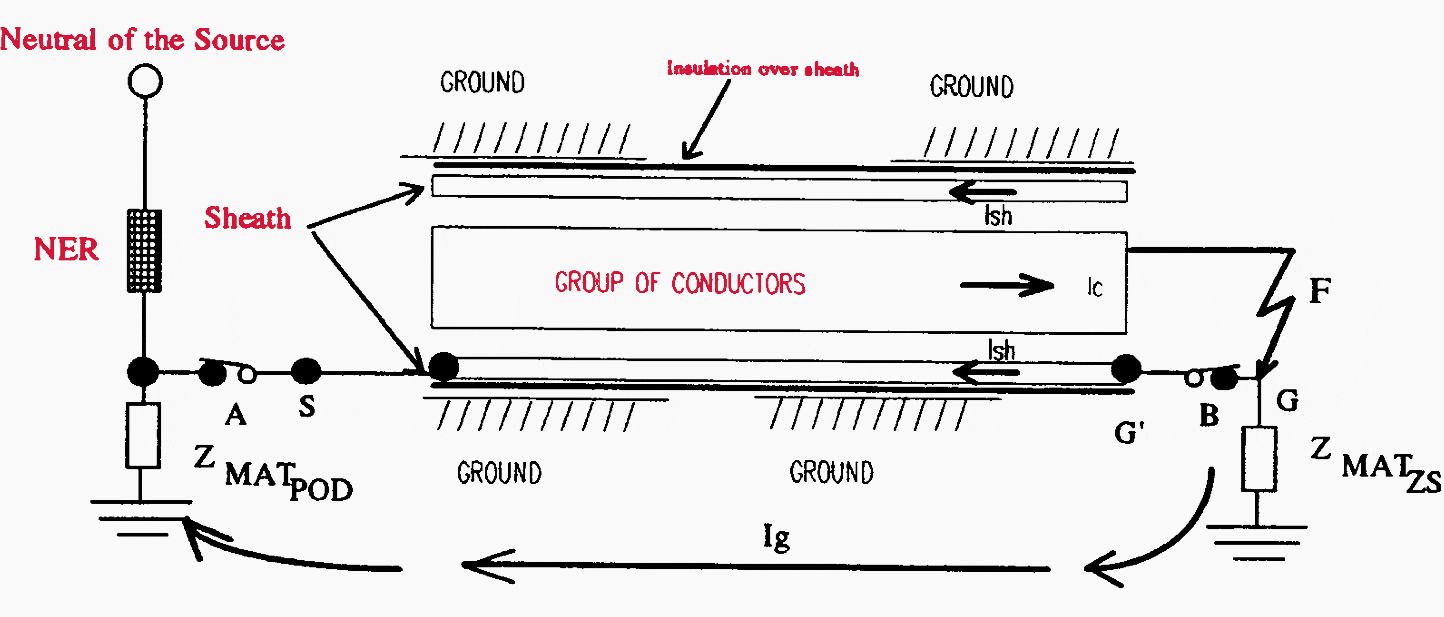Get access to premium HV/MV/LV technical articles, electrical guides & papers. Apply 20% discount code 99AF8 for a 1-year plan!

# Calculation of earth potential rise in the underground power distribution cable network

Home / Download Center / Electrical Engineering Books and Technical Guides / Electricity generation, t&d guides / Calculation of earth potential rise in the underground power distribution cable network

## Earth potential rise (EPR) calculation

The author has observed young graduate engineers using available computer programs to model substation earth mats and calculate earth potential rise (EPR) without understanding the importance of the parameters involved.

In one instance, an engineer was calculating EPR for a 33/11kV zone substation earth mat. The calculated earth mat resistance was 3 Ohms, the source substation had an 11 Ohms neutral earthing resister and the length of the 33kV line to the zone substation was approximately 10km.

This engineer used a 10kA fault current to simulate the substation earth potential rise and associated voltage contours. An engineer with some knowledge of power system fault analysis would have quickly worked out that the maximum phase to earth fault current (post transient steady state) at the source substation could not be greater than 33,000/(( 3 )(11 Ohm)) or 1732 amperes.

Obviously, the EPR of a 3 Ohm earth mat with 1732 A fault current is only 5196 Volts compared to 30kV calculated by the earth mat analysis software.An equivalent cable section. The cable is insulated from the ground. The conductors were grouped together to calculate equivalent GMR (Geometric Mean Radius of one conductor in mm) of 3 conductors.

The author, in early days of exposure to such calculations, also made similar mistakes. His mistakes were associated with calculation of EPR in an 11kV underground cable network. The author had fault analysis software (PSSU) which had the facility to calculate high impedance phase to earth fault currents. He used this facility and calculated earth fault currents and EPR in a cable network and it was a mistake.

The fault current calculated by PSSU was equal to the current in the faulty phase conductor and there was no way of finding out the proportions that returned to the source via the cable sheath and the general mass of earth. It was during these calculations the author realised that special considerations are required to calculate EPR in a cable network.

The fundamental part of calculation of the earth potential rise is the calculation of the phase to earth fault current magnitude in a given network. This requires network impedance calculations, fault current analysis and then design of the earthing system to assess and control the hazardous EPR.

The fault current analysis of a 3-phase 3-wire overhead line distribution network is relatively simple compared to that of an underground cable network, or a combination of overhead and underground networks.

In this paper, a method of calculating fault current split (between cable sheath and the general mass of earth), and the EPR and the transferred earth potential rise (TEPR) for the phase to earth faults in the overhead and the underground three core cable power distribution network will be presented.

 Title: Calculation of earth potential rise in the underground power distribution cable network  – Ashok Parsotam Format: PDF Size: 471 KB Pages: 45 Download: Right here | Get Download Updates | Get Premium Membership